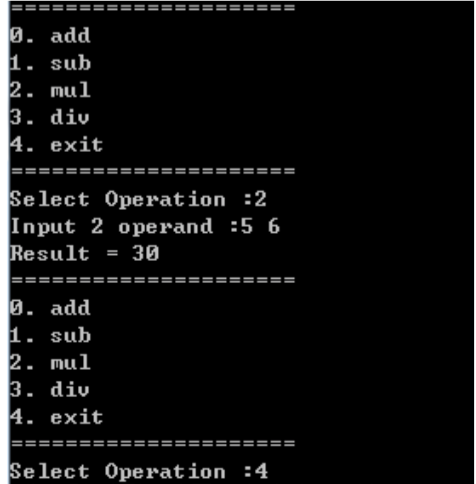int sub(int x, int y);
int mul(int x, int y);
int div(int x, int y);
int (*pf)(int, int) = { add, sub, mul, div };2个回答

`````` #include <iostream>
using namespace std;
return x+y;
}
int sub(int x,int y){
return x-y;
}
int mul(int x,int y){
return x*y;
}
int div(int x,int y){
return x/y;
}
int main(){
int (*pf)(int, int) = { add, sub, mul, div };
while(true){
cout<<"1. sub"<<endl;
cout<<"2. mul"<<endl;
cout<<"3. div"<<endl;
cout<<"4. exit"<<endl;

cout<<"Select Operation :";
int opeNum;
cin>>opeNum;
if(opeNum==4){
return 0;
}
cout<<"Input 2 operand :";
int a,b;
cin>>a>>b;
cout<<"Result = "<<pf[opeNum](a,b)<<endl;
}

}
``````

#include
#include

{
return a + b;
}
int sub(int a ,int b)
{
return a - b;
}
int mul(int a ,int b)
{
return a * b;
}
int dive(int a ,int b)
{
if (b == 0)
exit(1);
else
return a / b;
}
int (*pf)(int, int) = { add, sub, mul, dive };

int main()
{
int ch,x,y;
scanf("%d",&ch);
scanf("%d %d",&x,&y);
if (ch !=4)
printf("%d\n",(*pf[ch])(x,y));
else
exit(0);
return 0;
}